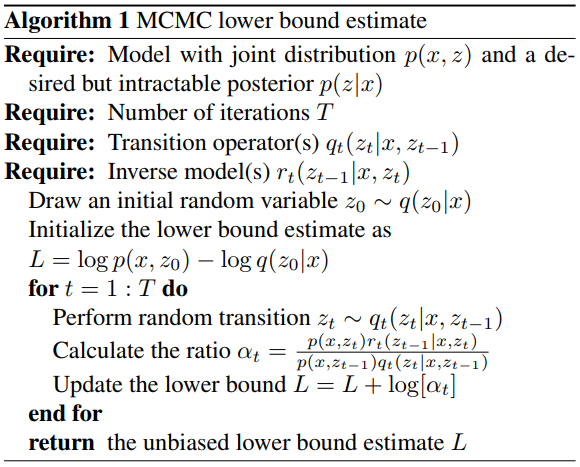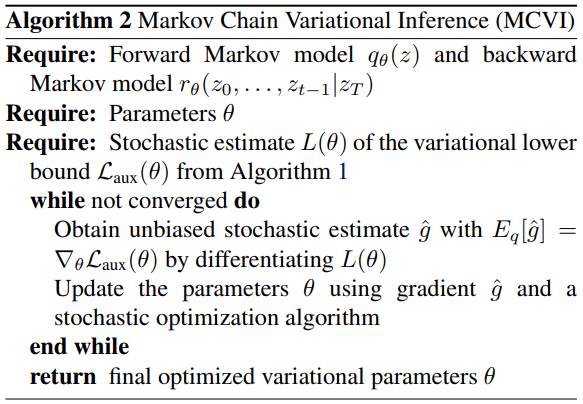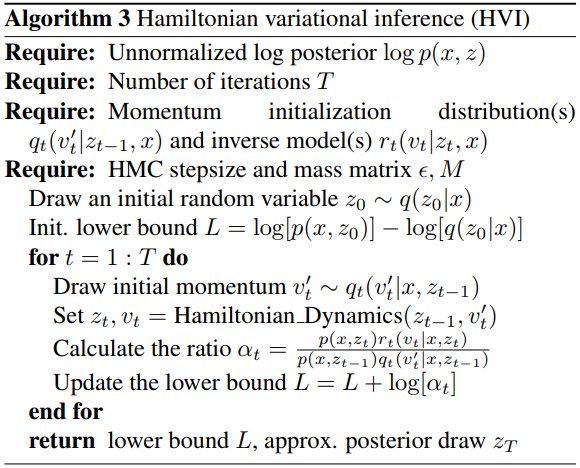## WHY?

Two approximation methods, Variational inference and MCMC, have different advantages: usually, variational inference is fast while MCMC is more accurate.

## Note

Markov Chain Monte Carlo (MCMC) is approximation method of estimating a variable. MCMC first sample a random draw $z_0$ and than draw a chain of variables from a stochastic transition operator q.

 It is proved that $z_T$ will ultimately converge to true posterior p(z x) with sufficiently enough samplings.

## WHAT?

Variational lowerbound contains posterior of latent variable q(z|x). This paper suggests to estimate q(z|x) more accurately with MCMC with auxiliary random variables and apply variational inference to ELBO with auxiliary variables. \

If we assume that auxiliary inference distribution also has a Markov structure,

Since auxiliary variational lowerbound cannot be calculated analytically, we estimate MCMC lowerbound by sampling from transitions($q_t$) and inverse model($r_t$).The gradient of resulted MCMC lowerbound can be calculated via remarameterization trick.One of the efficient methods of MCMC is Hamiltonian Monte Carlo (HMC). Hamiltonian MC introduce auxiliary variables v with the same dimension as z.## So?

Convolutional VAE with Hamiltonian Variational Inference (HVI) with 16 leapfrog step and 800 hidden nodes achieve slightly worse result than that of DRAW.

## Critic

Many MCMC algorithms variates can be used to improve the result.

Salimans, Tim, Diederik Kingma, and Max Welling. “Markov chain monte carlo and variational inference: Bridging the gap.” International Conference on Machine Learning. 2015.